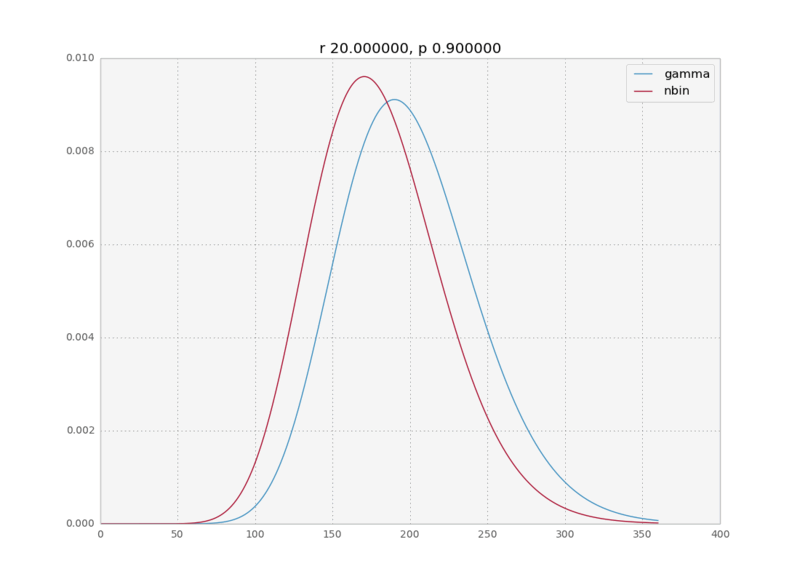# Gamma distribution approximation to the negative binomial distribution

In a recent data analysis project I was fitting a negative binomial distribution to some data when I realized that the gamma distribution was an equally good fit. And with equally good I mean the MLE fits were numerically indistinguishable. This intrigued me. In the internet I could find only a cryptic sentence on wikipedia saying the negative binomial is a discrete analog to the gamma and a paper talking about bounds on how closely the negative binomial approximates the gamma, but nobody really explains why this is the case. So here is a quick physicist’s derivation of the limit for large k.

First let’s define the distributions: the negative binomial is defined as

$$P(k)=\frac{\Gamma(k+r)}{k!\,\Gamma(r)} (1-p)^rp^k$$

with parameters $r,p$; and the gamma distribution is defined as

$$P(x)=\frac{\beta^{\alpha}}{\Gamma(\alpha)}x^{\alpha-1} e^{-\beta x}$$

with parameters $\alpha, \beta$.

We assume $p\rightarrow 1$, therefore $\langle k \rangle = \frac{pr}{1-p} \rightarrow \infty$; which means that the values of $k$ for which $P(k)$ is non negligible will be very high.Let’s start with the expression for the negative binomial and make the following replacement

$$\alpha=r, \beta=1-p$$

(I actually derived this ansatz from the numerical fits). We get the following expression:

$$P(k)=\frac{\beta^{\alpha}}{\Gamma(\alpha)} \frac{\Gamma(k+\alpha)}{k!} (1-\beta)^k$$

Let’s do stirling on both the denominator and numerator of the second term.

$$P(k)=\frac{\beta^{\alpha}}{\Gamma(\alpha)} \frac{(k+\alpha-1)^{k+\alpha-1} e^k}{e^{k+\alpha-1} k^k} (1-\beta)^k$$

Let’s split the term $(k+\alpha-1)^{k+\alpha-1} = (k+\alpha-1)^k(k+\alpha-1)^{\alpha-1}$. We can simplify the first term as $(k+\alpha-1)^k = k^k\left(1+\frac{\alpha-1}{k}\right)^k$. The second term is approximately $k^{\alpha-1}$ because $k»\alpha-1$. Now the $e^k$ and $k^k$ cancel out and we get:

$$P(k)=\frac{\beta^{\alpha}}{\Gamma(\alpha)} \frac{k^{\alpha-1} }{e^{\alpha-1}} \left(1+\frac{\alpha-1}{k}\right)^k (1-\beta)^k$$

Now, remember that

$$\lim_{n\rightarrow \infty} \left(1+\frac{x}{n}\right)^n = e^{x}$$

We can use this on the two last terms of the expression to obtain $e^{\alpha-1}e^{-\beta k}$, which yields the gamma expression we were looking for.